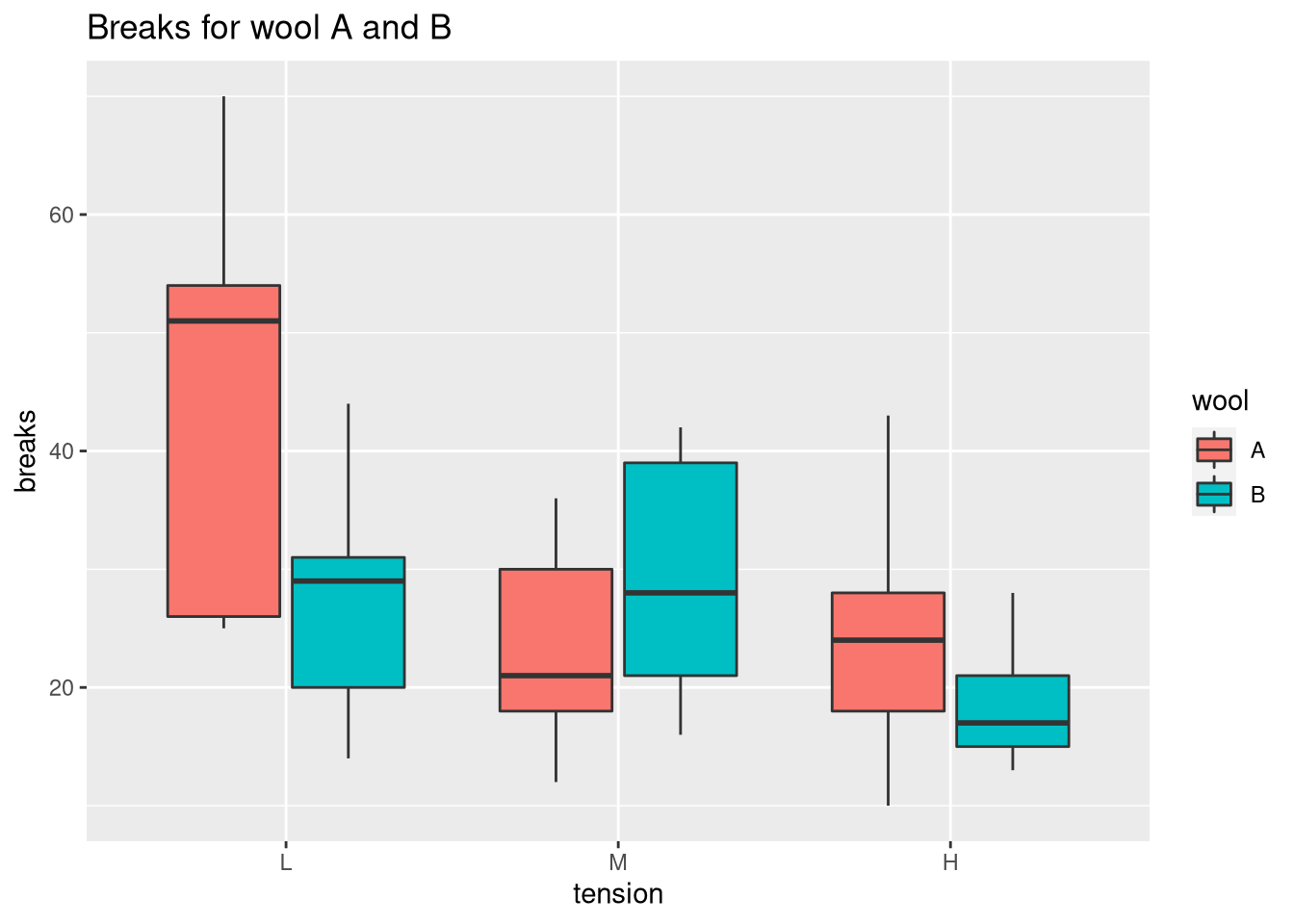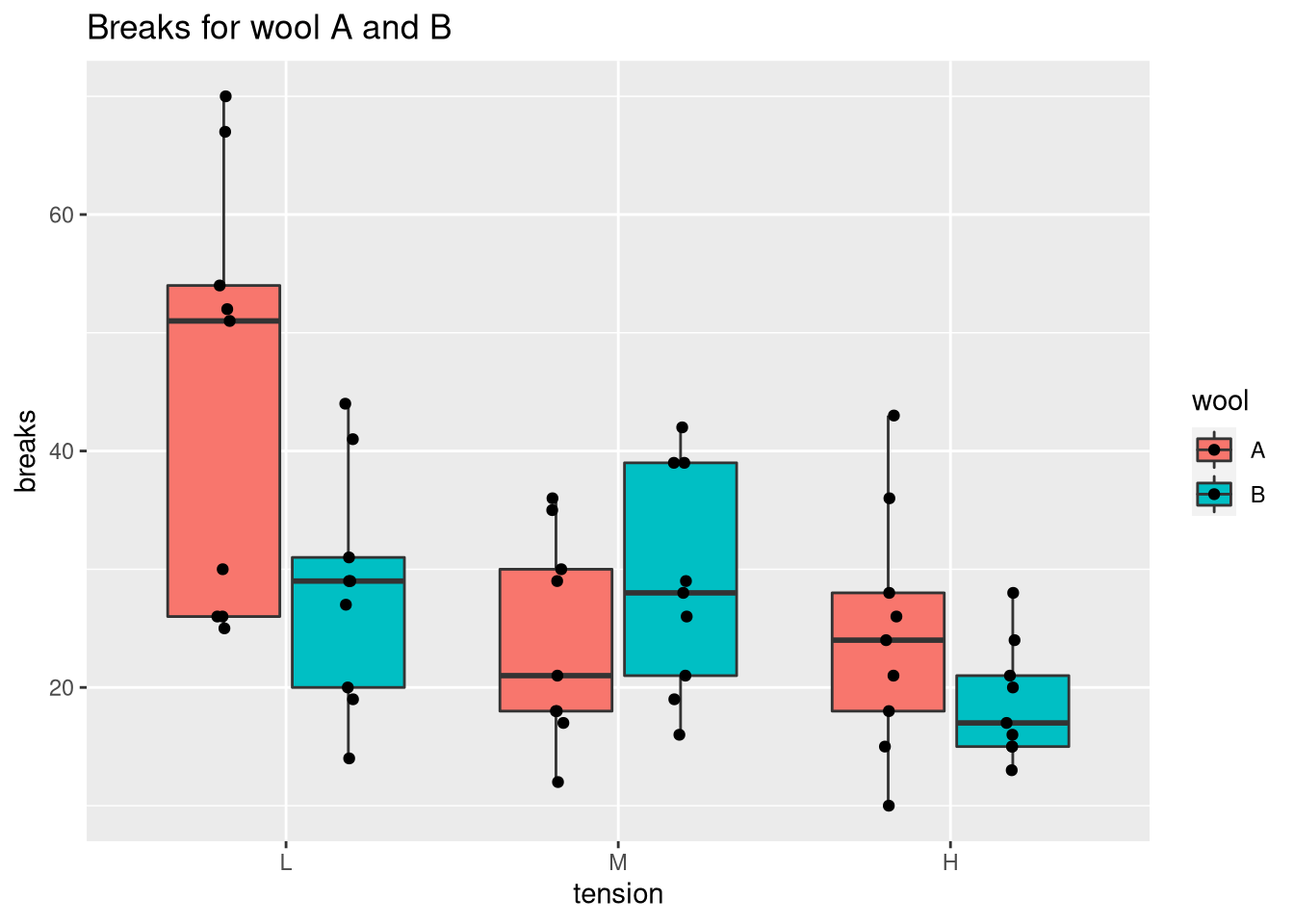[This article was first published on R on datascienceblog.net: R for Data Science, and kindly contributed to R-bloggers]. (You can report issue about the content on this page here)
Want to share your content on R-bloggers? click here if you have a blog, or here if you don't.

The box plot is useful for comparing the quartiles of quantitative variables. More specifically, lower and upper ends of a box (the hinges) are defined by the first (Q1) and third quartile (Q3). The median (Q2) is shown as a horizontal line within the box. Additionally, outliers are indicated by the whiskers of the boxes whose definition is implementation-dependent. For example, in `geom_boxplot` of ggplot2, whiskers are defined by the inter-quartile range (IQR = Q3 – Q1), extending no further than 1.5 * IQR.

## Creating a box plot in native R

We will use the warpbreaks data set to exemplify the use of box plots. In native R, a box plot can be obtained via `boxplot`.

```data(warpbreaks)
# create positions for tick marks, one more than number of bars
x <- warpbreaks\$breaks
# create labels
x.labels <- paste0(warpbreaks\$wool, "-", warpbreaks\$tension)
# specify colors for groups
group.cols <- c("darkred", "red", "darksalmon",
"darkblue", "blue", "lightblue")
cols <- c(rep(group.cols, 9), rep(group.cols, 9),
rep(group.cols, 9), rep(group.cols, 9),
rep(group.cols, 9), rep(group.cols, 9))
boxplot(x ~ warpbreaks\$wool + warpbreaks\$tension, col = group.cols)
legend("topright", legend = c(unique(x.labels)),
col = group.cols, pch = 20)```## Creating a box plot with ggplot

We could compare the tensions for each type of wool using `facet_wrap` in the following way:

```library(ggplot2)
ggplot(warpbreaks, aes(x = tension, y = breaks)) +
geom_boxplot() + facet_wrap(.~wool) +
ggtitle("Breaks for wool A and B")``````ggplot(warpbreaks, aes(x = tension, y = breaks, fill = wool)) +
geom_boxplot() +
ggtitle("Breaks for wool A and B")```### Showing all points

To view the individual measurements associated with the box plot, we set `outlier.shape = NA` to prevent duplicates and call `geom_point`.

```ggplot(warpbreaks, aes(x = tension, y = breaks, fill = wool)) +
geom_boxplot(outlier.shape = NA) +
ggtitle("Breaks for wool A and B") +
# dodge points horizontally (there are two bars per tick)
# and jitter points horizontally so that they don't overlap
geom_point(position = position_jitterdodge(jitter.width = 0.1))```Showing all the points helps us to identify whether the sample size is sufficient. In this case, most pairs of wool and tension exhibit high variabilities (especially wool A with tension L). Thus, the question would be whether this level of variability is inherent to the data or a result of the small number of samples (n = 9). Note that you can combine a box plot with a beeswarm plot to optimize the locations of the points.